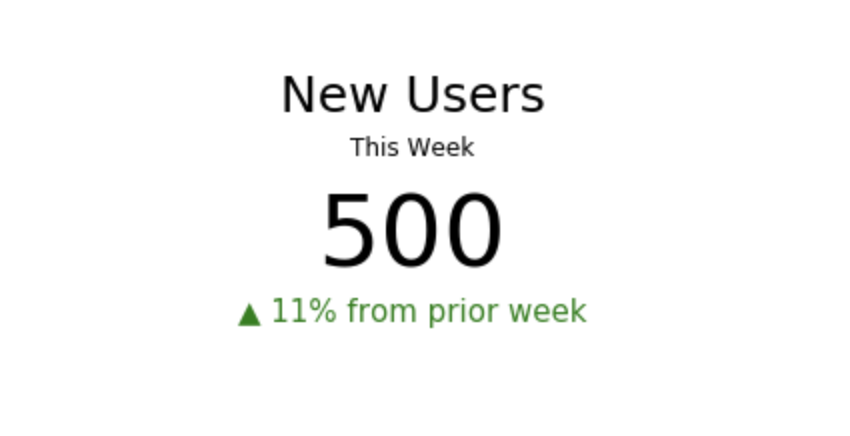# Chart Type - Number Overlays with Secondary Reference NumberContextualizing KPIs is a must. For instance, if a company has 500 users, how does that compare to the number of users last week? Using Periscope Data's Python Integration, we can create customized number overlays to cleanly present this information.

To create the visualization above, we assume that our SQL output generates 1 row of data with 2 columns:

1. Our KPI. in this case, number of users
2. The percent change (for proper formatting, use Periscope's percent formatter shortcut :%)

Then, we apply the following Python 3.6 code on the SQL output. Here we are also adding some helpful text to describe the KPI and adding some arrows and coloring to contextualize the KPI.

```import pandas as pd
import matplotlib.pyplot as plt

fig = plt.figure()
plt.axis('off')

plt.gcf().set_size_inches(8, 2)
plt.xticks([])
plt.yticks([])

if(df.iat[0,1]=='-'):
col='red'
dir="\u25bc"
val=df.iat[0,1][1:]
else:
col='green'
dir='\u25b2'
val=df.iat[0,1]

plt.text(.5, .95, 'New Users', fontsize=25, color='black', ha='center')
plt.text(.5, .75, 'This Week', fontsize=12, color='black', ha='center')
plt.text(.5, .25, str(df.iat[0,0]), fontsize=50, color='black', ha='center')
plt.text(.5, 0, dir + ' ' + val +' from prior week', fontsize=15, color=col, ha='center')

periscope.output(plt)
```

How does your business like to format KPIs in Number Overlays? Comment below!

5replies Oldest first
• Oldest first